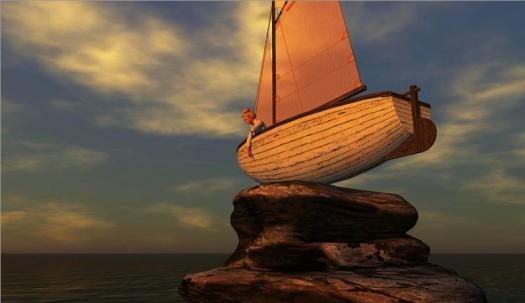# How Keen Is Your Perception?

Approved & Edited by ProProfs Editorial Team
At ProProfs Quizzes, our dedicated in-house team of experts takes pride in their work. With a sharp eye for detail, they meticulously review each quiz. This ensures that every quiz, taken by over 100 million users, meets our standards of accuracy, clarity, and engagement.
| Written by Salomeg
S
Salomeg
Community Contributor
Quizzes Created: 14 | Total Attempts: 72,040
Questions: 10 | Attempts: 3,514SettingsAre your eyes deceiving you or can you see the truth?

• 1.

### The red lines are:

• A.

Parallel and straight

• B.

Crooked

• C.

Unequal distance apart

• D.

Red

A. Parallel and straight
Explanation
The correct answer is "Parallel and straight" because the statement describes the red lines as being parallel to each other (not intersecting) and straight (not curved or bent).

Rate this question:

• 2.

### Are there gray squares in the white grid?

• A.

Yes.

• B.

No.

• C.

Maybe?

• D.

No, but there are some blue ones.

B. No.
Explanation
The answer is "No" because the question asks if there are gray squares in the white grid. It does not mention anything about blue squares. Therefore, the presence of blue squares does not affect the answer.

Rate this question:

• 3.

### The rows of black and white squares are:

• A.

Crooked.

• B.

Mismatched.

• C.

Parallel.

• D.

Gray.

C. Parallel.
Explanation
The rows of black and white squares are described as "Parallel" because they are running in the same direction and are equidistant from each other, forming straight lines.

Rate this question:

• 4.

### Look at the center circles. They are:

• A.

The same size

• B.

Left is bigger.

• C.

Right is bigger.

• D.

Equidistant

A. The same size
Explanation
The explanation for the correct answer is that the center circles are the same size. This means that they have equal dimensions and there is no difference in their sizes.

Rate this question:

• 5.

### The figures are:

• A.

The same size

• B.

The back one is largest

• C.

The middle is largest

• D.

The front one is largest

A. The same size
Explanation
The figures are all different sizes, but the correct answer is "the same size." This is because the statement "the same size" contradicts the information given about the sizes of the figures. Therefore, the correct answer is the opposite of what is stated.

Rate this question:

• 6.

### Regarding the blue lines:

• A.

Equal length

• B.

The right is longer than the left

• C.

The left is longer than the right

• D.

The center black line is longer than either.

A. Equal length
Explanation
The given answer states that the blue lines are of equal length. This means that both the left and right blue lines are the same length. It also suggests that the center black line is not longer than either of the blue lines. Therefore, all three lines are of equal length.

Rate this question:

• 7.

### How many colors do you see?

• A.

Four

• B.

Five

• C.

Two

• D.

Three

D. Three
Explanation
The question asks how many colors the person sees. Since the answer given is "Three," it implies that the person sees three different colors.

Rate this question:

• 8.

### The black square is

• A.

A rectangle

• B.

• C.

Actually a diamond

• D.

Perfectly straight

D. Perfectly straight
Explanation
The correct answer is "perfectly straight" because the question is asking about the characteristics of the black square. Out of the given options, "perfectly straight" is the only one that accurately describes the shape of a square, which has four equal sides that are straight and perpendicular to each other. The other options, such as "made of wavy lines," "actually a diamond," and "a rectangle," do not accurately describe the shape of a square.

Rate this question:

• 9.

### In which picture is the Leaning Tower of Pisa leaning more?

• A.

They're the same

• B.

It's leaning more in the left.

• C.

It's leaning more in the right.

• D.

It's not leaning.

A. They're the same
Explanation
The explanation for the given correct answer is that the statement "They're the same" means that the Leaning Tower of Pisa is not leaning more in any particular direction. This suggests that the tower is leaning at an equal angle towards both the left and right sides, resulting in a balanced leaning position. Therefore, there is no significant difference in the leaning angle between the left and right sides of the tower.

Rate this question:

• 10.

### What's going on here?

• A.

The gears are moving!

• B.

The gears aren't moving.

• C.

Only some of them are moving.

• D.

One of them is moving.Back to top